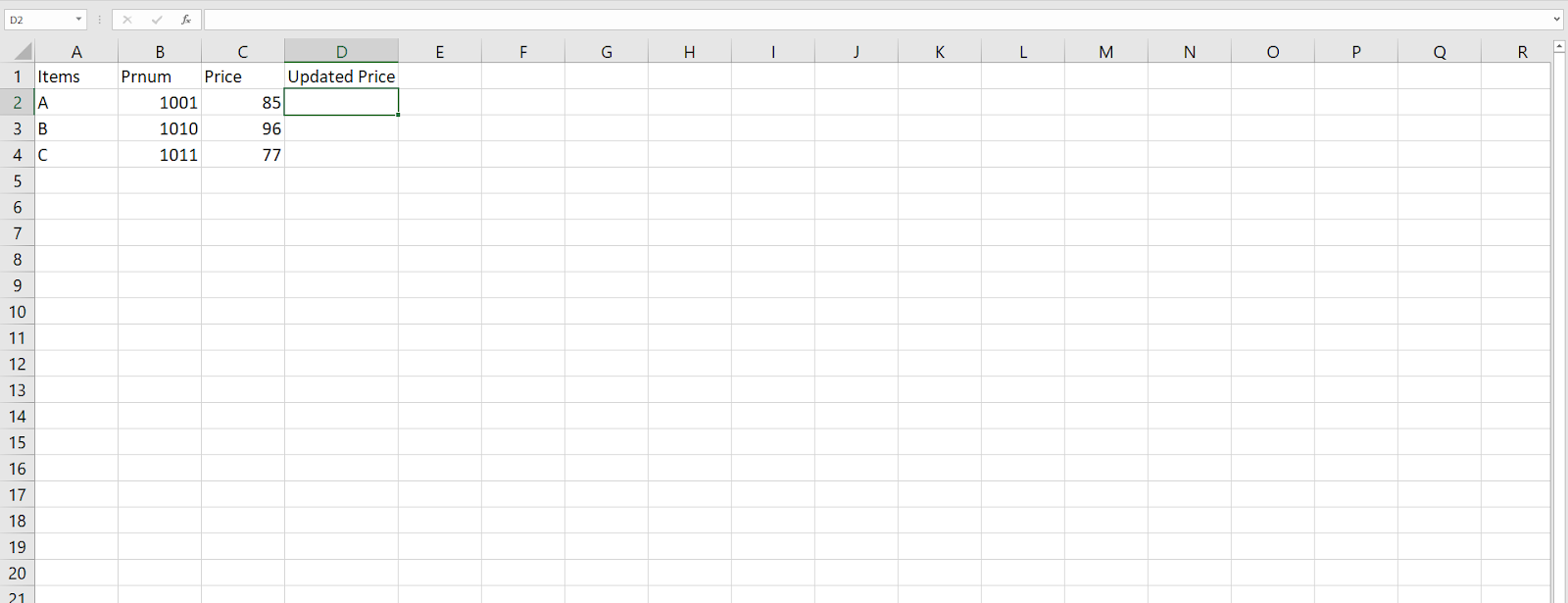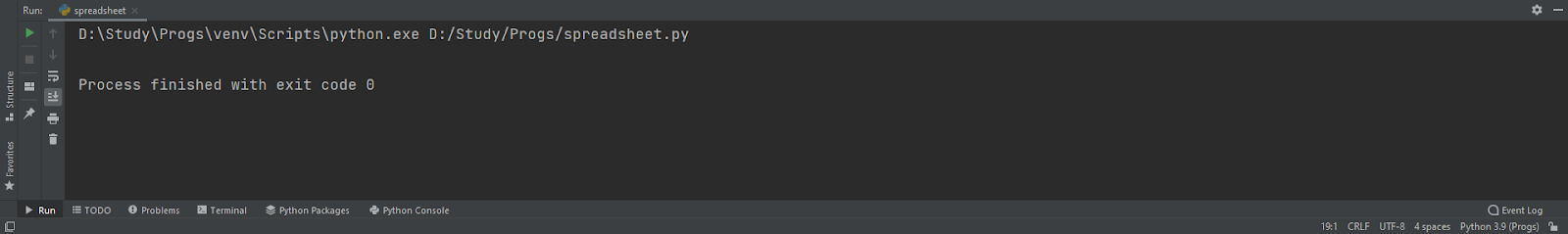# Automation in Python

## What is automation?

There are several tasks available that can be done easily with the help of feature automation in python. It refers to the process which takes place with the help of python codes or designing a program in python that does the work automatically. This saves a lot of time for the user and is more efficient and helps to overcome a lot of work in a less span of time.

Let us see an example to get the things cleared,

Here we will update an excel file with a new price with the help of python.

First, we need to design an excel sheet in which we will be working. Here we are taking an excel sheet with members as products and price. We will design a code in python that will return the updated price and a graph.Now let’s design a code which will return the update price and a graph.

### Explanation:

• We will install the openpyxl package. It can be done by typing it in the terminal of pycharm as: pip install openpyxl.

Where it has already been installed on my pc.

• For operating xl sheets in python we need to import this package or else the program will not run. The package is imported as: import openpyxl as xl , where xl will act as the object of the package.
• For the operation in graph we also need to import the graph package as: from openpyxl.chart import Barchart, Reference.
• Then we will design a separate function for the operation where we will receive the .xlsx file.
• We will load the file and the sheet in separate variables for working in the operation.
• Then we will start a loop from 2 to (max row+1). We are taking 2 as the values are stored in the file from the 2 rows and will go on to the (max row+1) i.e., till the last row getting incremented by 1 in every operation of the loop.
• Inside the loop we will provide the cell location with the value of the loop as row location and the default column location of this file as 3rd, where the value in the cell will get updated by multiplying with 5 and get stored in the 4th column.
• Then after the termination of the loop we will provide the values of the graph in the reference function with the values of the sheet the desired minimum row, maximum row and the desired minimum column add maximum column.
• The Barchart function is called, the values are added and the chart is then initialized to the sheet as the desired location.
• We save the workbook with a different file name as we don’t want to rewrite it in the same file.
• In the end the function is called with the file to run the operation.

Note: the excel sheet should be present inside the current running project in pycharm. We are using pycharm to run the python program.

## Example Of Python Automation:

```import openpyxl as xl
from openpyxl.chart import BarChart, Reference

sheet=wb['Sheet1']

for row in range(2, sheet.max_row+1):
cell=sheet.cell(row,3)
updatevalue=cell.value*5
updatedvalue_cell=sheet.cell(row,4)
updatedvalue_cell.value=updatevalue

values=Reference(sheet, min_row=2, max_row=sheet.max_row, min_col=4, max_col=4)
chart= BarChart()

wb.save('transaction_updated.xlsx')

auto_sheet('transaction.xlsx')```

Output:

The output of the program:The output of the updated excel sheet:

This is an example to demonstrate the process of automation in python. There are several other examples such as sending email, replying to email etc.

Here we come to the end of the topic. Hope we were able to explain the topic properly.